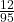## Lola pulls two marbles from a bag containing four red marbles, four blue marbles, and 12 yellow marbles without replacing them.

Question

Lola pulls two marbles from a bag containing four red marbles, four blue marbles, and 12 yellow marbles without replacing them.

What is the probability that she pulled out a red marble first and a yellow marble second? Express your answer in decimal form, rounded to the nearest hundredth.

0.09
0.13
0.25
0.32

in progress 0
3 weeks 2021-08-23T23:28:30+00:00 1 Answers 1 views 0

It’s the second option, 0.13.

Step-by-step explanation:

Step 1 : Find how many marbles there are in the bag all together.

4 + 4 + 12 = 20 marbles all together

Step 2 : Find what the probability of choosing a red marble is first.

4 red marbles out of 20 marbles =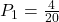Step 3 : Find the probability of choosing a yellow marble now.

Since one red marble was already taken, there are less marbles in total.

Instead of 20 marbles now there are only 19 marbles.

There are 12 yellow marbles out of 19 marbles =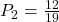Step 4 : To get the probability of both of these events happening, we multiply the 2 probabilities.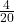·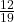=